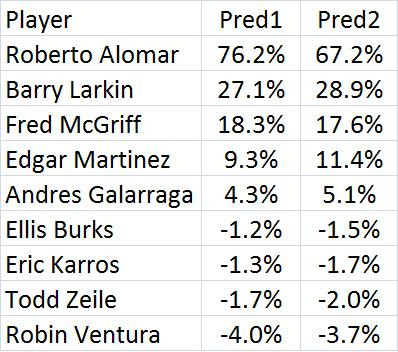## Thursday, December 17, 2009

### My Predictions For The Hall Of Fame Vote

I base my predictions on regression analysis of the voting from 1990-2009. I looked at voting in the first year of eligibility only. Here is the regression equation:

PCT = .04824*MVP + .45177*3000H + .16754*500HR + .00216*ASSQ10 - .00122*GGSQ7 + .04901*500SB
- .0119*WSIMPSQ50 + .09928*10000PA + .00112*WSAS + .06242*GGAS - .01282

I will explain what the variables mean below. The adjusted r-squared was .923. So 92.3% of the difference across players is explained by the equation. The standard error was .072. There were 181 players, all of those who came up for the first time from 1990-2009, except for Pete Rose. MVP is number of MVP awards won, 3000H is a dummy variable (1 if a player reached it, 0 otherwise). The 500HR is also a dummy variable as it is for 500SB and 10000PA (if you made it to 10,000 career plate appearances, you get a 1, 0 otherwise). I used all the voting data from 1990-2009.

What is ASSQ10? It is the square of the number of All-star games played in squared. But AS games played is maxed out at 10. The assumption here is that being an all-star has a positive exponential effect but only up to a point where no more games helps (I have a graph at a post last summer to help explain this-link below). The GGSQ7 is the same thing for Gold Gloves.

WSIMPSQ50 involves World Series play. First, WSIMP is World Series PAs times OPS. The idea here that the more you play in the World Series the more votes you would get, but by multiplying it by OPS, it also includes how well you played (or just hit). This gets maxed out at 50 and is squared, for the same reason as all-star games (yes, Reggie Jackson is first here and way ahead of everyone else at 141, with Dave Justice and Lonnie Smith tied for 2nd at 101).

The last two variables are interaction variables. GGAS is the gold glove variable multiplied by the all-star variable and WSAS is the world series variable times the all-star game variable. It looks strange that the coefficient values on GGSQ7 and WSIMPSQ50 are negative. But you might notice that they are positive on the interactive variables. I think this is like when a regression uses both X and X-squared in a regression if the phenomena is non-linear (an inverted parabola, for example). The coefficient on X ends up being positive while the x-squared coefficient is negative. The reason I put in these interactive variables was to see if players who were strong in both got an extra boost, as if there was some synergy going on. It seems like they did get an extra boost. My results in terms of r-squared and the standard error are better than what I got without the interaction variables last summer (links below).

All of the variables were significant at the 10% level except for WSIMP, which came close with a p-value of .13. The other variables all had p-values of under .05 with 7 under .01. I also divided the following variables by 1000 since the regression at first gave them a very low coefficient (due to them being very large numbers): WSIMPSQ50, WSAS and GGAS. With WSIMP50 going as high as 50 (then squared to get 2500) and AS going as high as 10 (then squared to get 100), the interaction term could be 250,000. Since the dependent variable can only go from 0 to 100, the coefficient would be very low (even thought the variables were significant). So I divided these three variables by 1000 (my stat package was showing coefficient values of .00000 before I did this).

So, what percentages does this equation predict for the first time eligibles once I plug in their own values? The table below shows this.Prediction1 is based on the above regression equation. I did another regression with the same variables but I took out Kirby Puckett and Mark McGwire. Puckett retired relatively early due to his eye problems and McGwire has the steroid scandal. Puckett got 82.1% of the vote in his first year of eligibility while the model predicts he would get 63.5%, for positive differential of 18.6%. McGwire got 23.5% of the vote his first time through while the model predicted him to get 40.3%, for a negative differential of -16.8%. Puckett had one of the biggest positive differentials while McGwire had one of the biggest on the negative side. I don't think any of the first-timers for 2010 are like Puckett or McGwire, so it might be reasonable to take them out. The predictions based on the model without those two guys is in the last column of the table above (and the standard error for that regression was .068). Things don't change much. But Alomar does slip below getting in. But that is still a high percentage and if he does not make it in the first time he probably will eventually.

I know that some predictions are negative. That is a drawback of this approach. The intercept is not terriblly negative (just -1.28%). So that is not a big problem. But GGSQ7 and WSIMPSQ50 do both have negative coefficients. So it is possible that a player might have gotten high scores there but if they could not get into any all-star games, those high scores would actually hurt them since the interaction variables would be zero and could not offset the negatives of the straight variables. But anyone with zero all-star games is probably not a Hall-of-Famer.

What Determines Vote Percentage In The First Year Of Hall Of Fame Eligibility? (Part 2)

What Determines Vote Percentage In The First Year Of Hall Of Fame Eligibility?